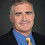## Thursday, November 10, 2022

### The Structure of Data and Machine Learning

Terry Tao entitled his 2006 Fields Medal Lecture "The Dichotomy between structure and randomness" and state the Structure Theorem: Every object is a superposition of structured object and a pseudorandom error. He gave many examples and how he used these results to prove (with Ben Green) that the primes contain arbitrarily long linear progressions.

There is a nice Kolmogorov interpretation. Recall K(x) is the smallest program that produces the string x. For a finite set S, K(S) is the smallest program that recognizes membership in S. For a string x, take the largest set S containing x such that K(x) is close to K(S) + log(|S|). S is the structure and x is a random element of S. Vereshchagin and Vitanyi have a nice paper on this topic.

Machine learning aims to discover the set S from a set of examples x. This is why I think of P = NP giving us an ideal machine learning algorithms--use P = NP to find a circuit that describes S for a time-bounded version of Kolmogorov complexity. Recent tools in machine learning seem to find this S without needing the full power of P = NP.

Consider languages where German or Japanese is a random example of a "natural language". Linguistics tries to understand the structure of natural languages. Recent ML translations algorithms use that structure (without understanding it) to translate between pairs of languages.

How about generative AI? Diffusion methods create a set S of all reasonable images by turning images into random noise. To create images it reverses that process, starting with random noise to create random elements of S. Prompts just add conditions to the process.

I read the Vereschagin-Vitanyi paper back in 2004 and the Kolmogorov structure model goes back to the 1970s. Finding the structure seemed intractable for any interesting application. Now that ML is proving this wrong, the world is a different place.

1.log(S) should be log(|S|)?

1.Fixed. Thanks.

2.If I understand it correctly, Lance means for one thing the following:
Many optimization problems are NP hard. Since the methods of ML (or some other extensions of ML) can be used to efficiently find solutions to these problems which are near optimal, or practically sufficiently optimal, it seems that P=NP is practically holds or practically credible rather than incredible.
To emphasize, it is so regardless of whether P=NP is used as assumption.

This puzzling phenomena might be explained without having to alter our beliefs about P vs NP.

In theory of computation, exact optimal solutions to NPC or NP hard optimization problems may not be in P; but practically optimal solutions to these problems may be in P (depending on how 'optimal' is meant)
And there may be gaps of infinity between the practically optimal and the exact optimal.
Also important to note is that for many practical optimization problems, it is difficult if possible to define what are their exact solutions.

By analogy, in physics, we are told that it is impossible for particles with nonzero static mass to be accelerated to the speed of light because infinite amount of energy is needed to achieve this; but it is possible for those particles to be accelerated near the speed of light.